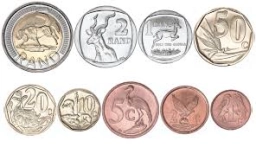# Christmas 81908

Markétka bought Christmas presents with a quarter of her savings. How much did she save if the gifts cost CZK 160? Express the rest of the savings as a fraction.

x =  640 Kc
y =  3/4

### Step-by-step explanation:Did you find an error or inaccuracy? Feel free to write us. Thank you!

Tips for related online calculators
Need help calculating sum, simplifying, or multiplying fractions? Try our fraction calculator.
Do you have a linear equation or system of equations and looking for its solution? Or do you have a quadratic equation?